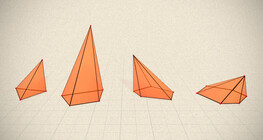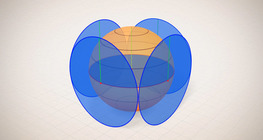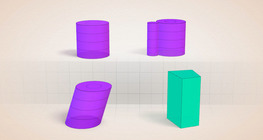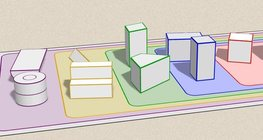Skaits: 0

Kopā: 0,00

0

# Perimetrs, laukums, virsmas laukums un tilpums

### Perimetrs, laukums, virsmas laukums un tilpums

Šajā animācijā iepazīstināsim ar formulām, kas ļauj aprēķināt figūru perimetru un laukumu, kā arī ģeometrisku ķermeņu virsmas laukumu un tilpumu.

Matemātika

Atslēgvārdi

Skaļums, virsma, apkārtmērs, platība, Lode, Piramīda, Cilindrs, apļa nozare, Zīmēt riņķi, Trīsstūris, taisnstūris, Kvadrāts, Konuss, Kuboīds, pamatplatība, sānu virsma, Paralelograms, Formula, ģeometrija, cietā ģeometrija, Matemātika

Saistītie vienumi

### Saistītie vienumi#### Cuboid

A cuboid is a polyhedron with six rectangular faces.#### Conic solids

This animation demonstrates various types of cones and pyramids.#### Cube

This animation demonstrates the components (vertices, edges, diagonals and faces) of the cube, one of the Platonic solids.#### Sphere

A sphere is the set of points which are all within the same distance from a given point in space.#### Conic sections

The conic section is a plane curve that is created when a right circular cone is intersected by a plane.#### Grouping of cuboids

This animation demonstrates various types of cuboids through everyday objects.#### Platonic solids

This animation demonstrates the five regular three-dimensional (or Platonic) solids, the best known of which is the cube.#### Ratio of volumes of similar solids

This 3D scene explains the correlation between the ratio of similarity and the ratio of volume of geometric solids.#### Regular square pyramid

A regular square pyramid is a right pyramid with a square base and four triangular faces.#### Surface area of spheres (demonstration)

The surface of a sphere consists of the set of points which are all at the same distance from a given point in space.#### Volume and surface area (exercise)

An exercise about the volume and surface area of solids generated from a ´base cube´.#### Volume of spheres (Cavalieri´s principle)

Calculating the volume of a sphere is possible using an appropriate cylinder and cone.#### Volume of spheres (demonstration)

The sum of the volume of the ´tetrahedrons´ gives an approximation of the volume of the sphere.#### Cylindrical solids

This animation demonstrates various types of cylindrical solids as well as their lateral surfaces.#### Grouping of solids

This animation demonstrates various groups of solids through examples.#### Net of a cube (exercises)

Not all nets consisting of 6 congruent squares are foldable into cubes.#### Solids of revolution

Rotating a geometric shape around a line within its geometric plane as an axis results in a solid of revolution.